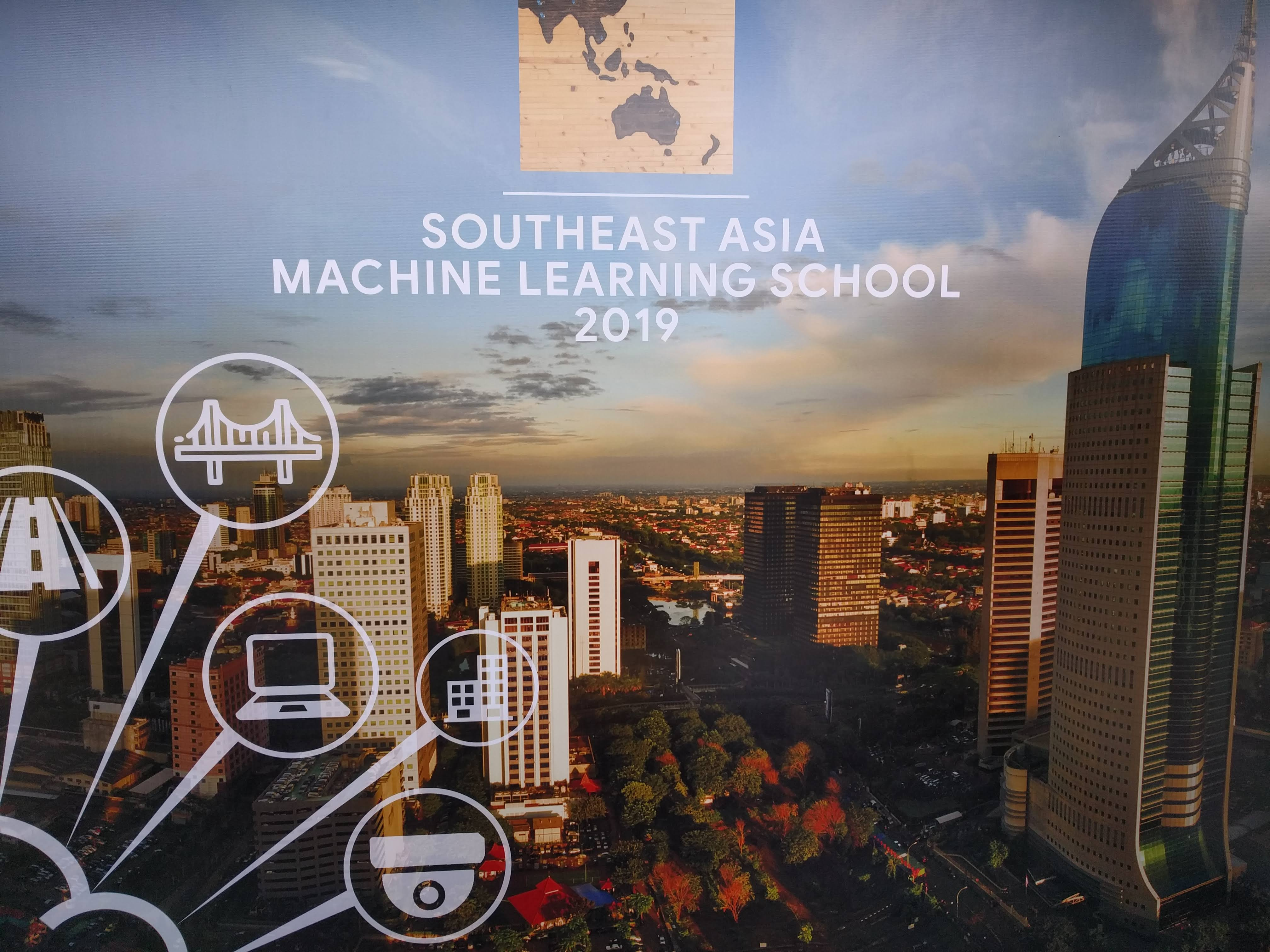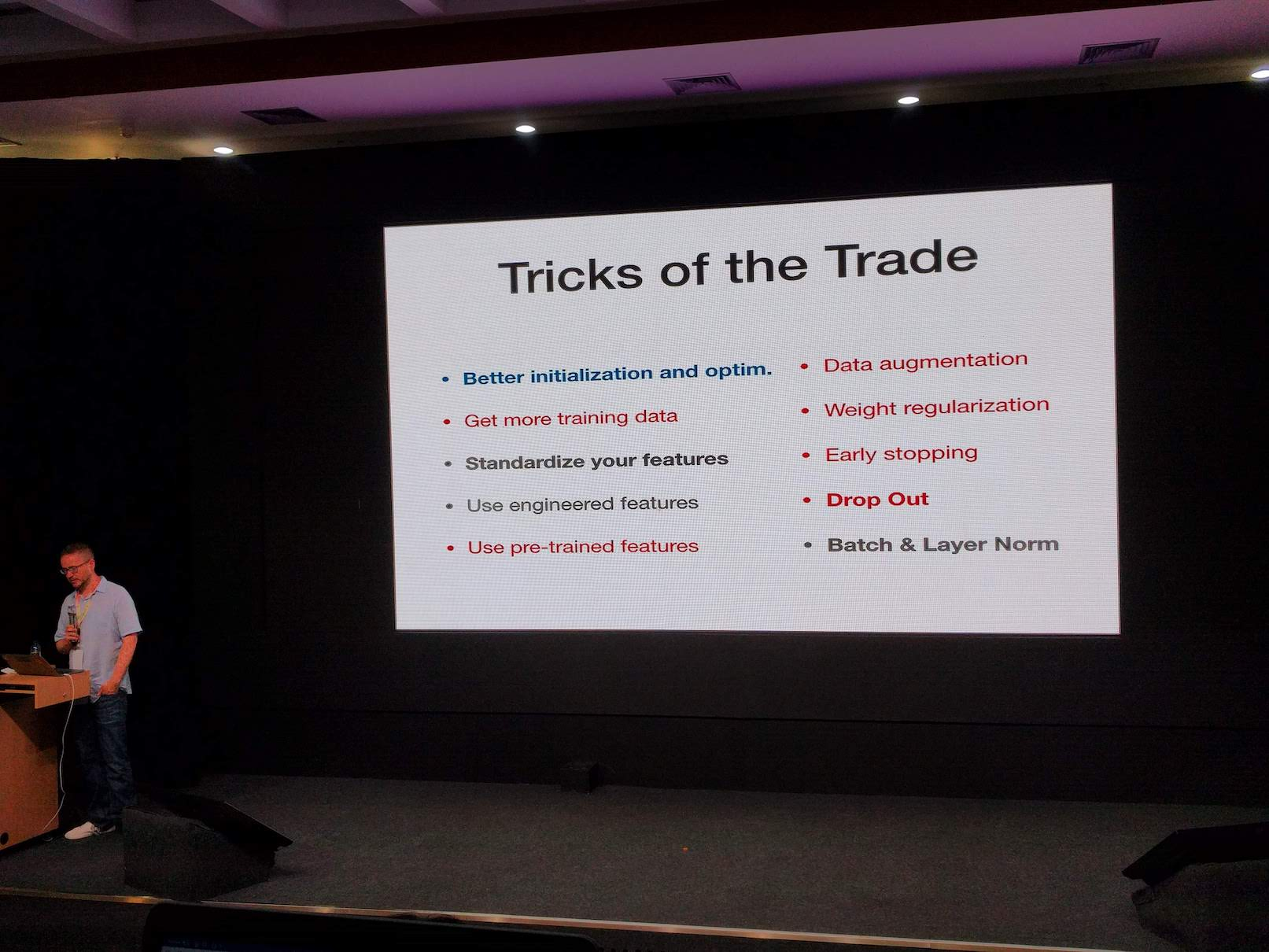# SEAMLS 2019 HighlightsAbout two weeks ago, I spent my time in Jakarta attending Southeast Asia Machine Learning School (SEAMLS) 2019. SEAMLS is a five-days event to learn the current state of the art in machine learning and deep learning. It aims to inspire, encourage, and educate more machine learning engineers, researchers, and data scientists within the Southeast Asia region. I am very fortunate to get selected from about 1,200 applicants. In this post, I will share few things I learned and caught my attention on each lecture.

## Day 1:

### Math foundation by Cheng Soon Ong [slides].

The first lecture focuses on the math foundation covering linear algebra, analytics geometry, matrix decomposition, vector calculus, probability and distribution, as well as continuous optimization.

I recommend Cheng Soon’s book to learn more details on math foundation in machine learning: https://mml-book.com/.

### Machine learning basics by Lee Wee Sun.

Lee Wee Sun delivers two lectures: introduction to machine learning [slides], and machine learning basics [slides]. In his first lecture, he briefly explains common machine learning problems: supervised learning, unsupervised learning, and reinforcement learning. He also presents some examples of machine learning tasks such as classification and regression, data compression, and generative models.

In his second lecture, he talks about the basic and fundamental concepts of machine learning:

1. loss function: we use loss function to help measure our success.
• Common loss function: 0-1 loss, square loss, and absolute loss.
• Empirical risk minimization
• Overfitting
• Regularization
• I.I.D Assumption
2. Maximum likelihood
• Maximum likelihood and minimizing empirical risk. For some distributions D, maximizing the log likelihood is equivalent to empirical risk minimization with appropriate loss functions.
• Maximum a posteriori (MAP) estimation
• Bayes estimation
• Unsupervised learning: density estimation, mixture model, autoregressive model
• Generative Adversarial Network (GAN)
3. Model selection
• validation set
• k-cross validation, leave-one-out cross validation
• what to do when learning fails
4. Feature selection and generation
• filter: mutual information, information gain
• wrapper: forward selection, backward elimination
• sparsity-inducing norms: l1 regularization, LASSO
• feature transformation and normalization: centering, unit range, standardization, clipping, sigmoidal transformation, logarithmic transformation, TF-IDF transformation
• dimension reduction: autoencoder, PCA

Some materials in his lecture are taken from few chapters in Understanding Machine Learning: From Theory to Algorithms by Shai Shalev-Shwartz and Shai Ben-David. I encourage you to read the book to understand fundamental theory underlying machine learning principles. The book is available here (free for personal use only).

### Practical session on Tensorflow and Google Colab by Subhodeep Moitra.

We have a practical session on the first day of SEAMLS. In this sesion, I learned about Seedbank, a cool website hosting a collection of interactive machine learning examples.

## Day 2:

### Simple unsupervised learning by Wray Buntine [slides].

We start second day with foundations of unsupervised learning. Wray talks about various aspects of unsupervised learning:

• Clustering
• How to build the cluster? How many clusters?
• Extension to clustering: hierarchical clustering, soft clustering, “multi-level” clustering (LDA), matrix factorization, multi-view tensor factorization
• What are the clusters used for?
• Typical uses of clustering: discovery tool, generative model, market segmentation, etc.
• Typical uses of matrix factorization: dimensionality reduction, summarization of relational content, recommender system, etc.
• Models and algorithms:
• Distance-based models: k-means, hierarchical algorithms
• Generative models
• Gaussian mixture model (GMM)
• Matrix factorization
• GMM algorithms:
• Greedy local search
• Gibbs sampling
• Variational method
• Foundations:
• Partition theory and label switching problem
• Cost function
• Evaluation

### Neural network basics (+tricks of the trade) by Chris Dyer [slides].

Chris begins his talk with examples of neural network applications, followed by explanations on why we favor neural networks.

He explains the details of feed-forward networks, bias and variance in neural networks, differentiable losses, learning optimization, minibatching, and implementation details. He also go through the details on computing derivatives including numeric differentiation (ND), symbolic differentiation (SD), and automatic differentiation (AD).

Second part of Chris’ talk is about tricks of the trade on working with neural networks. Neural network is powerful, but we have a lot of things to be tuned. He gives a general advice:

• Make sure you can overfit on a few examples
• Try to get a model that overfits on the training data (i.e. reduce bias)
• Then try to improve the overfit model (i.e. reduce the variance)Texts in blue: control bias. Texts in red: control variance. Texts in black: control both bias and variance. Texts in bold face discussed in-depth by Chris.

Optimization in neural network is hard because no guarantee about convergence in neural networks. But, we have few tricks:

• Make sure inputs and weights are statistically well-behaved. Input normalization allows you to use larger learning rates. Carefully scaling the variance during weight initialization can significantly help with learning.
• Use large numbers of parameters
• Use better optimizers
• Smarter update rules: momentum, RMSProp, Adam
• Better internal representation
• Dropout
• Batch and layer normalization

One interesting research question he asked during the talk: Does dropout increase or decrease interpretability?

## Day 3:

All talks on the third day are related to natural language processing.

### Sequence modeling and embeddings by Yun-Nung (Vivian) Chen [slides].

In this talk, Vivian explains the basic of word representation, word embeddings, and recurrent neural networks. In word representation, we want to capture the meaning of a word. There are two types of representations being used to represent meaning of a word. First type is knowledge-based representation such as wordnet. However, this approach requires laborious annotation effort, the annotation can be subjective, and newly-invented words need to be manually added to the knowledge-based. It is also difficult to compute word similarity in the knowledge base. Second type of representation leverages on available corpus (thus, it is called corpus-based representation). The representation can be atomic using one-hot representation or neighbor-based using SVD or word embeddings.

For word embeddings part, she explains more details on skip gram model, CBOW, and GloVe, as well as the evaluation of word embeddings.

In the last part of her talk, Vivian discuses about recurrent neural networks, LSTM, GRU, bidirectional RNN, and sequence prediction applications.

### Neural sequence generation by Kyunghyun Cho [slides].

I learned new types neural sequence generation beside the traditional unbounded autoregressive model. Kyunghyun introduces two models: iterative parallel decoding, and non-monotonic sequential generation. In iterative parallel decoding, the idea is that the decoder iteratively refine generated sequence (in denoising fashion). Tokens are generated in parallel instead of a word at a time in this framework.

In non-monotonic sequential generation framework, we don’t assume a pre-specified generation order (such as left to right in standard monotonic sequential generation). In this framework, a word is generated at an arbitrary position, then recursively generating its left and right following a binary tree.

Beside neural sequence generation, Kyunghyun Cho also discusses learning and inference on neural dialogue models.

### Practical session on Language Model by Rewon Child [slides].

Rewon gives practical on NLP using Tensorflow. We learned to implement a simple transformer model in this practical session.

## Day 4:

### Convolutional Neural Networks by Viorica Patraucean [slides].

Viorica introduces many aspects of convolutional neural networks (convnets):

• Convnets taxonomy
• Convnets inductive biases
• Hierarchical representation: abstraction increases with depth and size of receptive field
• locality of the data
• translation invariance
• Convolutional layer
• Pooling
• Strided convolutions and deconvolutions
• Initialization in convnets
• Other convolutions: 1×1 kernels, dilated convolutions, separable convolutions, grouped convolutions, dynamic lightweight convolutions

She also discusses many computer vision tasks including image classification, object detection, multiple objects detection, and semantic segmentation.

### Multi-modal Machine Learning by Douwe Kiela [slides].

Douwe gives an interesting talk discussing the meaning of multi-modal, multi-modal representation learning, multi-modal fusion, joint embedding spaces, and fusion by attention.

He also presents some applications on multi-modal machine learning: image captioning, visual question answering (VQA), visual reasoning, visual dialogue, and embodied QA.

### Practical session on CNNs by Mike Chrzanowski [slides].

In this practical session, Mike leads us to build a VGG-like convnet.

## Day 5:

### Deep probabilistic graphical models by Hung Bui [slides].

Hung gives a nice introduction to deep probabilistic graphical models. I suggest you to go through his slides if you are interested in this topics. He explains latent variable models, probabilistic graphical models, and their relation to deep probabilistic graphical models. He also covers training a deep probabilistic graphical model via variational inference, and reparameterization trick. At the end of his talk, he touches a bit on representation learning with deep probabilistic graphical models.

### Attention, self-attention, transformer, and BERT by Manaal Faruqui [slides].

Manaal introduces the basic of attention mechanism, then followed by self-attention, transformer architecture, and BERT. He also touches a bit on the interpretability of attention.

### Machine learning in Biomedicine by Truyen Tran [slides].

In the final lecture in SEAMLS, Truyen shows many applications of machine learning techniques in biomedicine domain. He presents various problems and applications on set, sequence, and graph data in biomedicine.

Beside lectures, there are three panel discussions and poster sessions. Overall, this event is well-organized, and I really like the program. The program covers good balance of fundamental and more advanced topics. Full programs are available here, along with the slides material. I highly recommend you to go through the slides material on topics you want to learn.## Author: Philips Kokoh

Philips Kokoh Prasetyo is a Principal Research Engineer at the Living Analytics Research Centre (LARC) in the Singapore Management University. He enjoys analyzing data from many different perspectives. His current interests include machine learning, natural language processing, text mining, and deep learning.